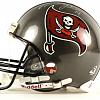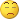# All John Ehlers Indicators... - page 452047

All fisher transform indicator

Files:898

tampa:
All fisher transform indicator

Thank you Tampafor nice indicators adding in to this thread!2047

All Inverse fisher transform indicator

Files:59

barnix:

Traders' Consortium > Rocket Science ....

Code for graph:

{ Synthetic cycle generator }

{ 5-wave synthetic cycle generator v4.0

Refresh chart for new simulations.

in MetaStock External Function DLLs folder.

For personal use only.

{ User inputs }

plot:=Input("Sine-wave cycles: Composite, Individual",1,2,1);

Sval1:=Input("Sine 1 value",-720,720,1);

Sval2:=Input("Sine 2 value",-720,720,3.5);

Sval3:=Input("Sine 3 value",-720,720,12);

Sval4:=Input("Sine 4 value",-720,720,42);

Sval5:=Input("Sine 5 value",-720,720,150);

{ Random generator engine }

x:=LastValue(

ExtFml("TMMath1.MyRand",C)/66.66667+.5);

{ Sine components }

sine1:=Sin(Cum(Sval1*x));

x:=LastValue(

ExtFml("Random.Number",-1)/66.66667+.5);

sine2:=Sin(Cum(Sval2*x))*.5;

x:=LastValue(

ExtFml("Random.Number",-1)/66.66667+.5);

sine3:=Sin(Cum(Sval3*x))*.25;

x:=LastValue(

ExtFml("Random.Number",-1)/66.66667+.5);

sine4:=Sin(Cum(Sval4*x))*.125;

x:=LastValue(

ExtFml("Random.Number",-1)/66.66667+.5);

sine5:=Sin(Cum(Sval5*x))*.0625;

{ Composite Sine wave }

composite:=sine1+sine2+sine3+sine4+sine5;

{ Plot in own window }

If(plot=1,composite,sine1);

If(plot=1,composite,sine2);

If(plot=1,composite,sine3);

If(plot=1,composite,sine4);

If(plot=1,composite,sine5)

### TMMath1.dll ?

the site is not working anymore273

Are you using Metastock? Why would you want to use this? Do you understand the code you just posted?59

sir

yes i use metastock also, and i wanted that dll to know, is this indicator usefull or not.273

debashis:
sir yes i use metastock also, and i wanted that dll to know, is this indicator usefull or not.

I am glad you have metastock, it is one of the classics. The answer to your question is no.2047

Files:26

Hi,

Ca anyone help me please ? Mr Ehler's new site is www.StockSpotter.com. On that site he shows the use of two indicators the "Mesa Cycle" and the "Mesa Momentum". Has anyone heard of these or knows if they are available in MT4 ? I have looked through this thread and they do not seem to have been posted with other names ?

Thanks

Nigel2973

Did you see the results of those too (this page : StockSpotter.com - Exit Signals ) There is only two tickers in profit out of all those in the list